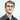# Advent of Code 2019 - Day 1

December 01, 2019

My approach to the advent of code 2019 problems, first year doing this and going to be using Go

## Day 1: The Tyranny of the Rocket Equation

### Part 1 - Fuel by mass

Fuel required to launch a given module is based on its mass. Specifically, to find the fuel required for a module, take its mass, divide by three, round down, and subtract 2.

First things first we want to implement a basic function to pull our input file into a Go structure, this makes our future work easier.

``````func readFileToArray(filename string) []string {
check(err)

lines := strings.Split(string(dat), "\n")
return lines
}``````

Next up, we need to calculate and return our total fuel required

``````func calcFuel(line int, sum int) int {
fuel := (line / 3) - 2
return fuel
}

func main() {
sum := 0
for _, x := range lines {
line, err := strconv.ParseInt(x, 10, 64)
if err == nil {
sum += calcFuel(int(line), sum)
}
}
fmt.Println(sum)
}``````

## Part 2 - Apparently, you forgot to include additional fuel for the fuel you just added.

Turns out, fuel has mass too! we need to add additional fuel for each unit of fuel we include.

I chose to go with a recursive approach, though a simple loop would work too.

``````func calcFuel(line int, sum int) int {
fuel := (line / 3) - 2

if fuel > 0 {
sum += fuel
sum = calcFuel(fuel, sum)
return sum
}

return sum
}

func main() {
sum := 0
for _, x := range lines {
line, err := strconv.ParseInt(x, 10, 64)
if err == nil {
sum = calcFuel(int(line), sum)
}
}
fmt.Println(sum)
}``````

Keep adding the smaller and smaller quantities of fuel to our total until the fuel amount <= 0

And thats it!

Github RepoWritten by Daniel Betteridge who lives and works in Perth, WA building useful things.